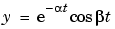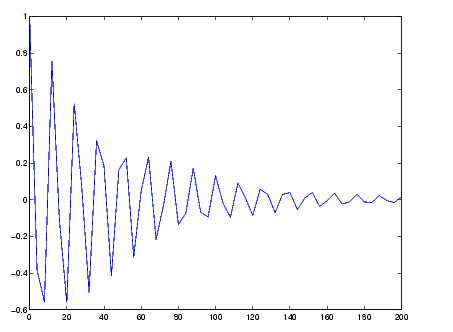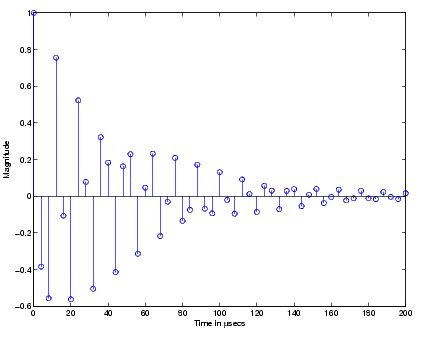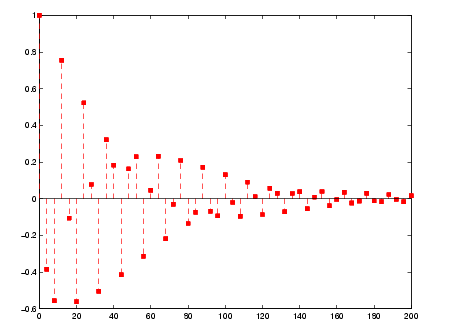GraphicsTwo-Dimensional Stem Plots

A stem plot displays data as lines (stems) terminated with a marker symbol at each data value. In a 2-D graph, stems extend from the x-axis.

The `stem` function displays two-dimensional discrete sequence data. For example, evaluating the functionwith the values

• ```alpha = .02; beta = .5; t = 0:4:200;
y = exp(-alpha*t).*cos(beta*t);
```

yields a vector of discrete values for `y` at given values of `t`. A line plot shows the data points connected with a straight line.

• ```plot(t,y)```

A stem plot of the same function plots only discrete points on the curve.

• ```stem(t,y)```

Add axes labels to the x- and y-axis.

• ``````xlabel('Time in \musecs')
```ylabel('Magnitude')
```

If you specify only one argument, the number of samples is equal to the length of that argument. In this example, the number of samples is a function of t, which contains 51 elements and determines the length of y.

Customizing the Graph

You can specify the line style, the type of marker, and the color used in the stem plot. For example, adding the string ':sr' specifies a dotted line (:), a square marker (`s`), and a red color (`r`). The `'fill'` argument colors the face of the marker.

• ```stem(t,y,'--sr','fill')```

Setting the aspect ratio of the x- and y-axis to 2:1 improves the utility of the graph. You can do this by setting the aspect ratio of the plot box using `pbaspect`.

• ````pbaspect`([2,1,1])
```

This is equivalent to setting the `PlotBoxApectRatio` property directly.

• ```set(gca,'PlotBoxAspectRatio',[2,1,1])
```

See `LineSpec` for a list of line styles and marker types.Discrete Data Graphs Combining Stem Plots with Line Plots© 1994-2005 The MathWorks, Inc.